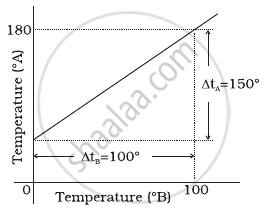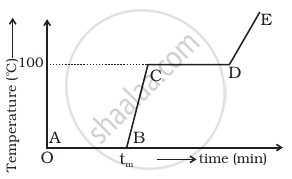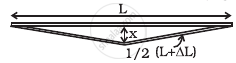# NCERT Exemplar solutions for Class 11 Physics chapter 11 - Thermal Properties of Matter [Latest edition]

#### Chapters## Solutions for Chapter 11: Thermal Properties of Matter

Below listed, you can find solutions for Chapter 11 of CBSE NCERT Exemplar for Class 11 Physics.

Exercises
Exercises [Pages 77 - 82]

### NCERT Exemplar solutions for Class 11 Physics Chapter 11 Thermal Properties of Matter Exercises [Pages 77 - 82]

#### MCQ I

Exercises | Q 11.1 | Page 77

A bimetallic strip is made of aluminium and steel (αAl > αsteel) . On heating, the strip will ______.

• remain straight.

• get twisted.

• will bend with aluminium on concave side.

• will bend with steel on concave side.

Exercises | Q 11.2 | Page 77

A uniform metallic rod rotates about its perpendicular bisector with constant angular speed. If it is heated uniformly to raise its temperature slightly ______.

• its speed of rotation increases.

• its speed of rotation decreases.

• its speed of rotation remains same.

• its speed increases because its moment of inertia increases.

Exercises | Q 11.3 | Page 77

The graph between two temperature scales A and B is shown in figure. Between upper fixed point and lower fixed point there are 150 equal division on scale A and 100 on scale B. The relationship for conversion between the two scales is given by ______.• (t_A - 180)/100 = t_B/150

• (t_A - 30)/150 = t_B/100

• (t_B - 180)/150 = t_A/100

• (t_B - 40)/100 = t_A/180

Exercises | Q 11.4 | Page 78

An aluminium sphere is dipped into water. Which of the following is true?

• Buoyancy will be less in water at 0°C than that in water at 4°C.

• Buoyancy will be more in water at 0°C than that in water at 4°C.

• Buoyancy in water at 0°C will be same as that in water at 4°C.

• Buoyancy may be more or less in water at 4°C depending on the radius of the sphere.

Exercises | Q 11.5 | Page 78

As the temperature is increased, the time period of a pendulum ______.

• increases as its effective length increases even though its centre of mass still remains at the centre of the bob.

• decreases as its effective length increases even though its centre of mass still remains at the centre of the bob.

• increases as its effective length increases due to shifting of centre of mass below the centre of the bob.

• decreases as its effective length remains same but the centre of mass shifts above the centre of the bob.

Exercises | Q 11.6 | Page 78

Heat is associated with ______.

• kinetic energy of random motion of molecules.

• kinetic energy of orderly motion of molecules.

• total kinetic energy of random and orderly motion of molecules.

• kinetic energy of random motion in some cases and kinetic energy of orderly motion in other.

Exercises | Q 11.7 | Page 79

The radius of a metal sphere at room temperature T is R, and the coefficient of linear expansion of the metal is α. The sphere is heated a little by a temperature ∆T so that its new temperature is T + ∆T. The increase in the volume of the sphere is approximately ______.

• 2πRα∆T

• πR2α∆T

• 4πR3α∆T/3

• 4πR3α∆T

Exercises | Q 11.8 | Page 79

A sphere, a cube and a thin circular plate, all of the same material and same mass are initially heated to same high temperature.

• Plate will cool fastest and cube the slowest

• Sphere will cool fastest and cube the slowest

• Plate will cool fastest and sphere the slowest

• Cube will cool fastest and plate the slowest

#### MCQ II

Exercises | Q 11.9 | Page 79

Mark the correct options:

1. A system X is in thermal equilibrium with Y but not with Z. System Y and Z may be in thermal equilibrium with each other.
2. A system X is in thermal equilibrium with Y but not with Z. Systems Y and Z are not in thermal equilibrium with each other.
3. A system X is neither in thermal equilibrium with Y nor with Z. The systems Y and Z must be in thermal equilibrium with each other.
4. A system X is neither in thermal equilibrium with Y nor with Z. The system Y and Z may be in thermal equilibrium with each other.
Exercises | Q 11.10 | Page 79

‘Gulab Jamuns’ (assumed to be spherical) are to be heated in an oven. They are available in two sizes, one twice bigger (in radius) than the other. Pizzas (assumed to be discs) are also to be heated in oven. They are also in two sizes, one twice big (in radius) than the other. All four are put together to be heated to oven temperature. Choose the correct option from the following:

1. Both size gulab jamuns will get heated in the same time.
2. Smaller gulab jamuns are heated before bigger ones.
3. Smaller pizzas are heated before bigger ones.
4. Bigger pizzas are heated before smaller ones.
Exercises | Q 11.11 | Page 80

Refer to the plot of temperature versus time (figure) showing the changes in the state of ice on heating (not to scale).1. The region AB represents ice and water in thermal equilibrium.
2. At B water starts boiling.
3. At C all the water gets converted into steam.
4. C to D represents water and steam in equilibrium at boiling point.
Exercises | Q 11.12 | Page 80

A glass full of hot milk is poured on the table. It begins to cool gradually. Which of the following is correct?

1. The rate of cooling is constant till milk attains the temperature of the surrounding.
2. The temperature of milk falls off exponentially with time.
3. While cooling, there is a flow of heat from milk to the surrounding as well as from surrounding to the milk but the net flow of heat is from milk to the surounding and that is why it cools.
4. All three phenomenon, conduction, convection and radiation are responsible for the loss of heat from milk to the surroundings.

#### VSA

Exercises | Q 11.13 | Page 80

Is the bulb of a thermometer made of diathermic or adiabatic wall?

Exercises | Q 11.14 | Page 80

A student records the initial length l, change in temperature ∆T and change in length ∆l of a rod as follows:

 S.No. l(m) ∆T (C) ∆l (m) 1. 2 10 4 xx 10^-4 2. 1 10 4 xx 10^-4 3. 2 20 2 xx 10^-4 4. 3 10 6 xx 10^-4

If the first observation is correct, what can you say about observations 2, 3 and 4.

Exercises | Q 11.15 | Page 81

Why does a metal bar appear hotter than a wooden bar at the same temperature? Equivalently it also appears cooler than wooden bar if they are both colder than room temperature.

Exercises | Q 11.16 | Page 81

Calculate the temperature which has same numeral value on celsius and Fahrenheit scale.

Exercises | Q 11.17 | Page 81

These days people use steel utensils with copper bottom. This is supposed to be good for uniform heating of food. Explain this effect using the fact that copper is the better conductor.

#### SA

Exercises | Q 11.18 | Page 81

Find out the increase in moment of inertia I of a uniform rod (coefficient of linear expansion α) about its perpendicular bisector when its temperature is slightly increased by ∆T.

Exercises | Q 11.19 | Page 81

During summers in India, one of the common practice to keep cool is to make ice balls of crushed ice, dip it in flavoured sugar syrup and sip it. For this a stick is inserted into crushed ice and is squeezed in the palm to make it into the ball. Equivalently in winter, in those areas where it snows, people make snow balls and throw around. Explain the formation of ball out of crushed ice or snow in the light of P–T diagram of water.

Exercises | Q 11.20 | Page 81

100 g of water is supercooled to –10°C. At this point, due to some disturbance mechanised or otherwise some of it suddenly freezes to ice. What will be the temperature of the resultant mixture and how much mass would freeze?

[S_w = 1cal/g/^circC and L_(Fusion)^w = 80cal/g]

Exercises | Q 11.21 | Page 81

One day in the morning, Ramesh filled up 1/3 bucket of hot water from geyser, to take bath. Remaining 2/3 was to be filled by cold water (at room temperature) to bring mixture to a comfortable temperature. Suddenly Ramesh had to attend to something which would take some times, say 5-10 minutes before he could take bath. Now he had two options: (i) fill the remaining bucket completely by cold water and then attend to the work, (ii) first attend to the work and fill the remaining bucket just before taking bath. Which option do you think would have kept water warmer? Explain.

#### LA

Exercises | Q 11.22 | Page 82

We would like to prepare a scale whose length does not change with temperature. It is proposed to prepare a unit scale of this type whose length remains, say 10 cm. We can use a bimetallic strip made of brass and iron each of different length whose length (both components) would change in such a way that difference between their lengths remain constant. If αiron = 1.2 × 10−5/K and αbrass = 1.8 × 10−5/K, what should we take as length of each strip?

Exercises | Q 11.23 | Page 82

We would like to make a vessel whose volume does not change with temperature (take a hint from the problem above). We can use brass and iron (β_(vbrass) = (6 xx 10^(–5))/K and β_(viron) = (3.55 xx 10^(–5))/K) to create a volume of 100 cc. How do you think you can achieve this.

Exercises | Q 11.24 | Page 82

Calculate the stress developed inside a tooth cavity filled with copper when hot tea at temperature of 57°C is drunk. You can take body (tooth) temperature to be 37°C and α = 1.7 × 10–5/°C, bulk modulus for copper = 140 × 109 N/m2.

Exercises | Q 11.25 | Page 82

A rail track made of steel having length 10 m is clamped on a raillway line at its two ends (figure). On a summer day due to rise in temperature by 20° C, it is deformed as shown in figure. Find x (displacement of the centre) if αsteel = 1.2 × 10–5/°C.Exercises | Q 11.26 | Page 82

A thin rod having length L0 at 0°C and coefficient of linear expansion α has its two ends maintained at temperatures θ1 and θ2, respectively. Find its new length.

Exercises | Q 11.27 | Page 82

According to Stefan’s law of radiation, a black body radiates energy σT4 from its unit surface area every second where T is the surface temperature of the black body and σ = 5.67 × 10–8 W/m2K4 is known as Stefan’s constant. A nuclear weapon may be thought of as a ball of radius 0.5 m. When detonated, it reaches temperature of 106 K and can be treated as a black body.

1. Estimate the power it radiates.
2. If surrounding has water at 30°C, how much water can 10% of the energy produced evaporate in 1s?  [Sw = 4186.0 J/kg K and Lv = 22.6 × 105 J/kg]
3. If all this energy U is in the form of radiation, corresponding momentum is p = U/c. How much momentum per unit time does it impart on unit area at a distance of 1 km?

## Solutions for Chapter 11: Thermal Properties of Matter

Exercises## NCERT Exemplar solutions for Class 11 Physics chapter 11 - Thermal Properties of Matter

Shaalaa.com has the CBSE Mathematics Class 11 Physics CBSE solutions in a manner that help students grasp basic concepts better and faster. The detailed, step-by-step solutions will help you understand the concepts better and clarify any confusion. NCERT Exemplar solutions for Mathematics Class 11 Physics CBSE 11 (Thermal Properties of Matter) include all questions with answers and detailed explanations. This will clear students' doubts about questions and improve their application skills while preparing for board exams.

Further, we at Shaalaa.com provide such solutions so students can prepare for written exams. NCERT Exemplar textbook solutions can be a core help for self-study and provide excellent self-help guidance for students.

Concepts covered in Class 11 Physics chapter 11 Thermal Properties of Matter are Anomalous Expansion of Water, Measurement of Temperature, Ideal-gas Equation and Absolute Temperature, Thermal Expansion, Calorimetry, Change of State - Latent Heat Capacity, Conduction, Convection, Radiation, Newton’s Law of Cooling, Qualitative Ideas of Black Body Radiation, Wien's Displacement Law, Stefan's Law, Liquids and Gases, Thermal Expansion of Solids, Green House Effect, Specific Heat Capacity, Heat and Temperature.

Using NCERT Exemplar Class 11 Physics solutions Thermal Properties of Matter exercise by students is an easy way to prepare for the exams, as they involve solutions arranged chapter-wise and also page-wise. The questions involved in NCERT Exemplar Solutions are essential questions that can be asked in the final exam. Maximum CBSE Class 11 Physics students prefer NCERT Exemplar Textbook Solutions to score more in exams.

Get the free view of Chapter 11, Thermal Properties of Matter Class 11 Physics additional questions for Mathematics Class 11 Physics CBSE, and you can use Shaalaa.com to keep it handy for your exam preparation.